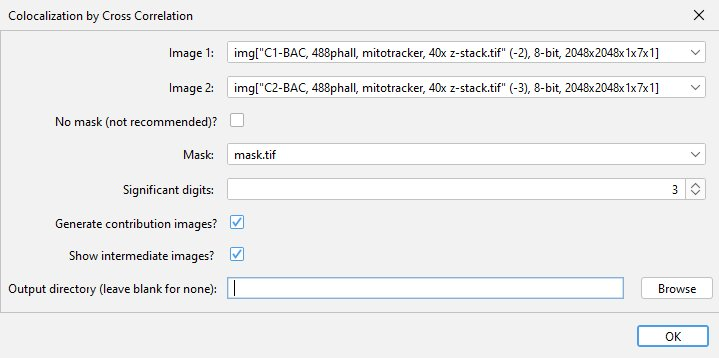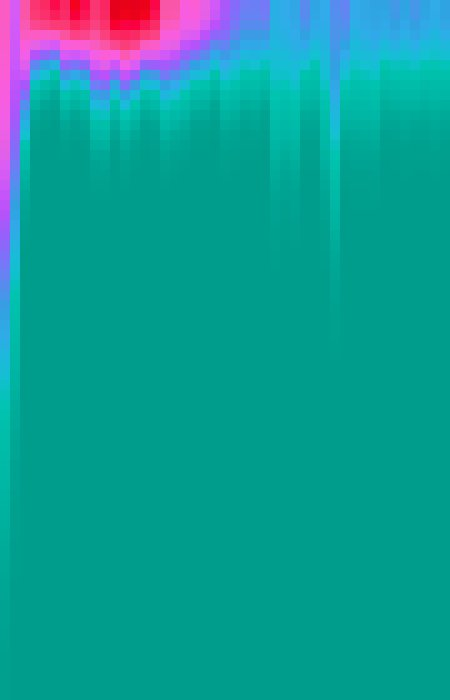# Colocalization by Cross Correlation

## Colocalization by Cross Correlation

This plugin attempts to determine: the average distance between non-randomly spatially associated particles, the standard deviation of that distance (which should also reflect the width of the PSF in the image for diffraction limited images), and a statistical measure of confidence of the association. It does this by performing a cross-correlation function (CCF) between the two images, operating in a similar manner to Van Steensel’s CCF except this plugin performs the CCF in all directions and provides additional information, such as a confidence level. It currently works on 2D/3D single-channel images, supports time-series analysis, and requires a mask of all possible localizations for the signal in one of the images.

## Installing the Plugin:

Available on the list of ImageJ updates sites. Requires Fiji.

## How to use Colocalization by Cross Correlation:

### Prepare a mask for the Costes randomization

It’s best to use an appropriate background subtraction method on the two data images in order to lower the background pixel values. While pixels outside of the masked region do not contribute to the cross-correlation result, having a high signal to background ratio within the masked region will help improve the confidence signifiantly. Additionally, the images (particularly 3D) need to be correctly scaled (Image > Properties), otherwise all axes will be assumed to have the same scale and your mean correlation distance will be in pixels.

### Run the pluginThe plugin can be found in the Analyze > Colocalization menu after it has been installed. At the dialog menu, select the two images and the randomization mask to be used. Image 1 is the image that will be randomized and requires an appropriate mask. If the possible localizations for your dye/stain encompasses the entire image, you can check the no mask box instead. For the Costes’ randomization cycles count, you can typically input a low number (5-8), however if you have sparse signal within a large mask volume you will probably want to do more cycles. If the “Show intermediate images” box is checked, the plugin will open images showing the correlations (before and after subtraction of random associations), and a sample randomization image. This can be useful for understanding the function of the plugin, or for visualizing the direction of the correlation if your sample has a polarized axis. More details on the intermediate images can be found below.

## Interpreting the results:

To help describe the results, we will use the simple, idealized example shown below. This example analyzes the cross-correlation of two 2D images composed of equally sized dots (shown as a composite image). Most of these dots are paired to dots of the other channel at a set distance, however some are not at this distance or are not paired. We can see from the results that the strongly correlated dots are approximately 44 pixels apart, with a standard deviation of ~5.5 pixels (for actual microscope data, this distance would be in scaled units instead of pixels). The contribution image highlights the dots that are around this range of each other. We used correlation at a distance for this example to highlight one of the strengths of this technique, but this can be done for traditional “colocalized” images that have a mean cross-correlation distance near zero.

Here’s a detailed description of each of the result windows:

### Correlation plot

A radial profile plot will be displayed, it contains the radial profile of the original cross-correlation image (blue line), the radial profile of the cross-correlation after subtraction of random associations (green line), and a Gaussian curve fit to the subtracted profile (red dots). The distance between the blue line and the green line is a visual indicator of the confidence value described below. The Y-axis is the average of the cross-correlation function. While not technically arbitrary, it is most easily viewed as a measure of relative correlation. The range of the graph is set automatically to fit the Gaussian curve. If you wish to view all the data select More > Set Range to Fit all…

### Gaussian fit analysis

This text window will contain (in scaled units), the mean distance for the discovered correlation, and the standard deviation of that correlation (sigma), as well as an estimation of confidence for this correlation (range 0-100). The confidence is determined by taking the area under the curve (AUC) of the subtracted correlation radial profile (in range of mean ± 3×sigma) divided by the AUC of the original correlation radial profile (in same range). Values closer to 100 indicate a strong likelihood of true correlation. Values near zero indicate low to no correlation between the two images. I currently estimate that values of ~20 or greater indicate some degree of correlation (within the range specified by the Gaussian curve), though this is with very limited data. Confidence values can be affected by many things, including image noise and background, an inappropriate randomization mask, and optical aberrations.

#### Contribution of each image to the gaussian fit

Two new images will be created that display the signal from each analyzed image that contributed to the cross-correlation and gaussian fit result. It is important to note that this image is always generated and the data it contains will always be visible, even if you do NOT have a strong correlation between the two images. The pixel intensity values should NOT be used as an indicator of overall correlation between the images (that’s what the confidence value is for), but the relative brightness within an image can be used as an approximate indicator of how strongly that particular signal contributed to the correlation result. This relative brightness indication can be easily seen in the example data above: In our original data, all the dots are of the same size and intensity, however, in the resulting contribution images, the dots that remain are varied in their brightness based on how much they contributed to the cross-correlation result (you’ll notice that the brightest dots are all oriented in the same direction).

## Working with time-series data:

Working with time-series data is not that different than working with non-time-series data. Every frame of your data is analyzed individually, in the exact same manner that non-time-series data would be analyzed. Thus, all inputs (including the mask) must have the same number of frames. The output generated from the plugin has been changed to better suit time-series data:Heat map of gaussian fit: An example of the heat map generated with time-series data, shown with the Ice lookup table. Each column of pixels shows the gaussian curve fit to the cross-correlation for a single frame.

The most noteable difference with time-series data is how the data for the correlation plot is displayed. Instead of line-graphs, the plugin generates heat maps, where the x-axis is time, the y-axis is distance with 0 at the top, and the intensity is the average of the cross-correlation function at that distance. As we cannot simultaneously show the data for the three lines that would be shown in the correlation plot when using a heat map, we instead generate a three channel image. The first channel (defaults to red), displays the gaussian curve fit to the profile of correlation after subtraction of random associations, equivalent to the red dots from the correlation plot. The second channel (defaults to green) shows the cross-correlation function results after subtraction of random associations, equivalent to the green line from the correlation plot. And the third channel (defaults to blue) show the results of the original cross-correlation function, equivalent to the blue line from the correlation plot.

Another change with time-series data is that in addition to the gaussian fit analysis text window, which now displays the fit data for the frame with the highest correlation, the plugin will output a table of gaussian fit results, showing the gaussian fits and confidence for each frame. To save this table, go to File > Export > Table…, click browse and save the file as a .csv file (you must add the extension or you will get an error message).

Lastly, the contribution images will still be generated and are functionally the same as for their non-time series counterparts. However, it’s important to note that the contribution is evaluted on a per frame basis, and thus each frame shows the signal contribution to the gaussian fit results for that frame. Thus, if your cross-correlation distance shifts over the course of an experiment, the contribution images will display this shifting cross-correlation.

## How it works & intermediate images description:

First, this plugin applies the provided mask to both images, setting any pixels outside the masked region to zero. If this is not done, these pixels contribute to the original cross-correlation result, but this contribution is then always subtracted out during the process described below, leading to a decrease in confidence. After applying the mask, this plugin then performs an initial cross-correlation to create a original cross-correlation image. Bright regions in the cross-correlation image correspond to a high correlation between the images when the second image is shifted by a vector equal to the distance form the center of the cross-correlation image to the bright region. Thus, if you see a bright spot in the cross-correlation image that is 2 µm to the left of the image center, it means their is a positive correlation between image 1 and image2 when image2 is shited left 2 µm.

To remove the contribution from potential larger repeating structures (such as cells and nuclei), and to correct for correlation that results from high signal density, a second cross-correlation image generated from randomized images is then subtracted from the original correlation image. This occurs through cycles of:

1. Randomizing Image1 using the Costes randomization method and the mask of all theoretically possible localizations for the signal in Image1 (i.e. a mask of the cells, or the nuclei), which is provided by the user.

2. Calculating the cross-correlation of the randomized image with Image2, then averaging this over successive cycles.

After the subtraction process, we generate a radial profile of the subtracted data, showing the correlation between the images at different offsets, then fit a gaussian curve to it. We also generate a radial profile for the original correlation data before subtraction, as this is needed to establish a measure of confidence. The confidence is calculated as the area under the curve (AUC) of the subtracted correlation radial profile (in the range of mean ± 3×sigma) divided by the AUC of the original correlation radial profile (in same range) as a percentage. The confidence value, along with the mean and sigma of the gaussian fit are displayed in a log window. Higher values of confidence, closer to 100, indicate that two images likely have a true spatial correlation at the indicated distance.

To generate the contribution images, we further modify the subtracted cross-correlation image, by effectively multiplying it with the gaussian fit in order to create a cross-correlation image that only retains the data within our gaussian curve range. This gaussian-modified cross-correlation image is then used to back-calculate the contribution images. Image1Contribution = (image2 ∗ gaussModifiedCorr) × image1. Image2Contribution = (image1 ★ gaussModifiedCorr) × image2. Key: ∗ -> convolve, ★ -> correlate.